# SSC Reasoning Ability Practice Questions (Day-4)

Dear Aspirants, Here we have given the Important SSC CGL Exams 2018 Practice Test Papers. Candidates, those who are preparing for SSC CGL Exams 2018 can practice these questions to get more confidence to Crack SSC CGL Exams 2018 Examination.

[WpProQuiz 4082]

1) In a row of students, Manu is 7th from the bottom end of row.  Shreya is 10th ranks above Manu. If Shrey is 8th from the top end, then how many students are there in this row?

a) 25

b) 26

c) 24

d) 23

2) 7 yr ago, the age of Sahil was equal to the present age of Nihal. Sum of Sahil’s age 5 yr ago and Nihal’s age 6 yr later is 58 yr. If Ruchi is 4 year elder to Sahil, then what will be Ruchi’s age (in yr) after 10 yr?

a) 38 yr

b) 46 yr

c) 42 yr

d) 36 yr

3) If ‘α’ denotes ‘subtracted from’, ‘β’ denotes ‘multiplied by’, ‘θ’ denotes “added to” and ‘δ’ denotes “divided by”, then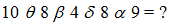a) 7

b) 5

c) 9

d) 11

4) If 92 A 42 B 32 = 56 and 72 A 22 B 12 = 44, then 112 A 52 B 72 = ?

a) 29

b) 32

c) 47

d) 24

5) In each of the following question below are given some statements followed by some conclusions. Taking the given statements to be true even if they seem to be at variance from commonly known facts, read all the conclusions and then decide which of the given conclusion logically follows the given statements?

Statements:

Some girls are clever.

All clever are hardworking.

Conclusions:

I) Some girls are not hardworking.

II) Some girls are hardworking.

III) Some hardworking are not girls.

a) Conclusions I and II follow

b) Only conclusion II follow.

c)  Conclusions I and III follow

d) All conclusions follow.

6) From the given options, which answer figure can be formed by folding the figure given in the question?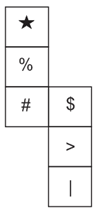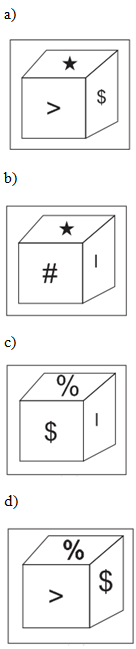7) If QUICKLY is coded as OSGAIJW. Then how will HUE be coded as?

a) ZMA

b) FSC

c) HZK

d) HNI

8) A piece of paper is folded and punched as shown below in the question figures. From the given answer figures, indicate how it will appear when opened.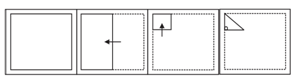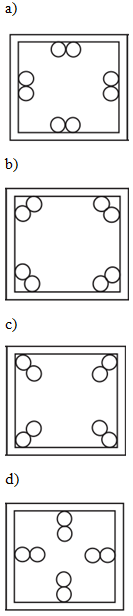9) Select the missing number from the given responses.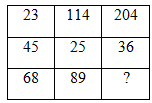a) 157

b) 168

c) 21

d) 15

10) If a mirror is placed on the line MN, then which of the answer figures is the right image of the given figure? Question figures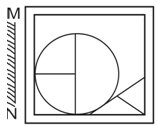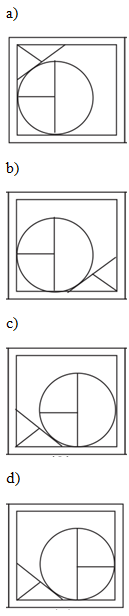11) A word is represented by only one set of numbers as given in any one of the alternatives. The sets of numbers given in the alternatives are represented by two classes of alphabets as shown in the given two matrices. The columns and rows of Matrix – I and numbered from 0 to 4 and that of Matrix – II are numbered from 5 to 9. A letter from these matrices can be represented first by its row and next by its column, for example, ‘K’ can be represented by 20, 34 etc., and ‘Z’ can be represented by 67, 88 etc. Similarly, you have to identify the set for the word ‘PURE’.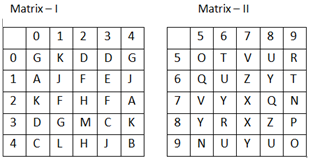a) 89, 96, 86, 13

b) 34, 34, 56, 79

c) 41, 44, 67, 96

d) 0, 10, 79, 57

Directions (Q 12-13): A series is given with one term missing. Select the correct alternative from the given ones that will complete the series.

12) ACE, FHJ, KMO, ?

a) QRS

b) PRT

c) PRU

d) QRV

13) 9, 18, 72, 576, ?

a) 8116

b) 8216

c) 9016

d) 9216

14) In the following question, select the number which can be placed at the sign of question mark (?) from the given alternatives.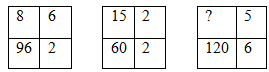a) 3

b) 4

c) 8

d) 14

15) A word is represented by only one set of numbers as given in any one of the alternatives. The set of numbers given in the alternatives are represented by two classes of alphabets as shown in the given two matrices. The columns and rows of Matrix – I are numbered from 0 to 4 and that of Matrix – II are numbered from 5 to 9. A letter from these matrices can be represented first by its row and next by its column, for example, ‘R’ can be represented by 23, 31, etc., and ‘0’ can be represented by 75, 98 etc. Similarly, you have to identify the set of the word ‘TRAY’.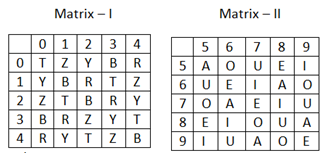a) 00, 04, 68, 02

b) 21, 41, 97, 41

c) 34, 12, 55, 11

d) 42, 23, 89, 23

Total number of students in the row

= (7 + 10+ 8) –1 = 25 – 1 = 24

Let the present age of Sahil = x yr

Present age of Ruchi = (x + 4) yr

According to the question,

x – 7 = 4

x – y = 7

And (x – 5) + ( y + 6) = 58

x + y = 58 – 1

x + y = 57

On solving Eq (i) and (ii), x = 32 yr

Ruchi’s age after 10 yr = (x + 4) + 10

= 32 + 4 + 10 = 46 yr

3) Answer: d)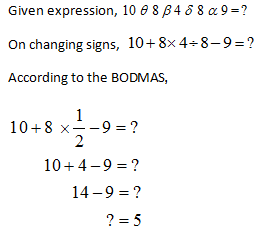As, 92 A 42 B 32

(9)2 – (42 + 32) = 81 – (16 + 9) =56

And 72 A 22 B 12

(7)2 – (22 + 12) = 49 – (4+1) = 44

Similarly,

112 A 52 B72  (11)2 – (52 + 72)

= 121 – (25 + 49) = 47

According to the statements,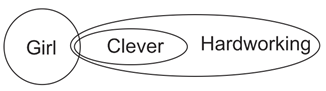Hence, only conclusion II follows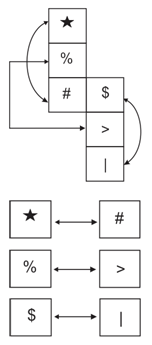Since the opposite surface can never be seen in a cube. Hence, answer figure (1) Can be formed by folding the question figure.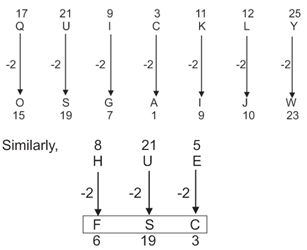Hence, HUE will be coded FSC in that language.

On opening a folded and punched paper it will appear as answer figure (1).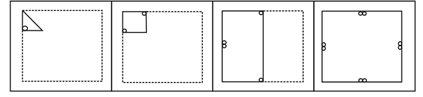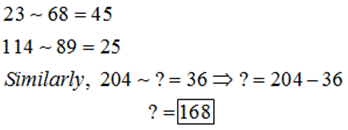On placing the mirror on line MN question figure will appear as answer figure (4).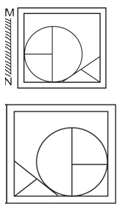According to the question,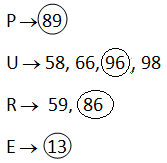Hence, PURE => 89, 96, 86, 13

Order of series is as follows.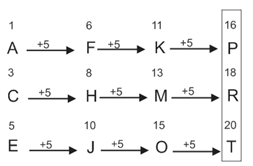Hence, ‘PRT’ will come in place of question mark (?).

Order of series is as follows.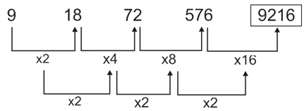Hence, number 9216 will come in place of question mark (?).

As,       8 x 6 x 2 = 96

And 15 x 2 x 2 = 60

Similarly, ? x 5 x 6 = 120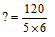According to the question,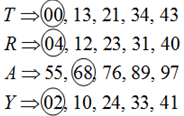Hence, TRAY =>00, 04, 68, 02

*********************************

### SSC Reasoning Ability Practice Questions – (Day-1)